# Cash Conversion Cycle

One metric that companies use to assess effective cash flow management is the cash conversion cycle (CCC).

The Cash flow of a company can be analogous to its life bloodline. Efficient cash flow generation and management are critical to the success of an enterprise in conducting its daily operations, pursuing investing opportunities, and meeting financial obligations.

Poor management of cash flow can lead to inability to meet payments, increasing the probability of financial distress and bankruptcy.

Cash Conversion Cycle is defined as the length of time (in days) needed to transform inventory purchases into actual cash receipts. It takes into consideration the company’s time commitment towards collecting receivables and paying its suppliers, and is an important measure of a company’s internal liquidity.

The CCC can be calculated as the sum of the inventory conversion period, receivables conversion period, and the parables conversion period.

CALCULATION STEPS

Firms typically follow a working capital cycle, whereby the acquisition of inventory is stored for a certain period of time, and subsequently sold, thus converting such purchases into sales and ultimately cash. This is precisely what the cash conversion cycle represents.

As a formula,

Days in Inventory Outstanding (DIO) + Days in Sales Outstanding (DSO) – Daysin Payales Outstanding = Cash Conversion Cycle

Calculating the CCC can be done in 4 steps, and requires information from the 3 main working capital accounts: Inventory, Accounts Receivable, and Accounts Payable. Note that certain income statement items are needed as well.

Assume the following:

 Net Revenue \$40,000 Inventory \$1,000.00 Cost of Goods Sold \$25,000.00 Average Receivables \$850 Average Payables \$2,500 Cycle Period (Days) 365

STEP 1: DETERMINE THE DAYS IN INVENTORY OUTSTANDING (DIO)

First we calculate the Inventory Turnover – defined as the number of times inventory is sold in a year: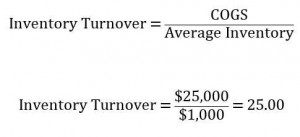Then calculate the Inventory Conversion Period, which is the average time it takes a firm to convert inventory purchases into sales: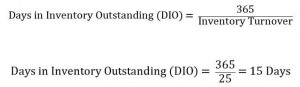STEP 2: DETERMINE THE DAYS IN SALES OUTSTANDING (OR DSO)

First find the Receivables Turnover – the average number of times that receivables are turned over (or collected):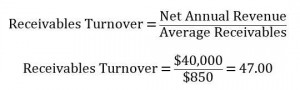Then find the Receivables Conversion Period (DSO), which measures the average time it takes to convert receivables into actual cash receipts.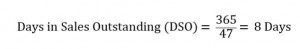STEP 3: DETERMINE THE DAYS IN PAYABLE OUTSTANDING (DPO)

Calculate the Payables Turnover Ratio,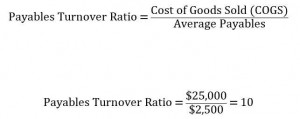Followed by the payables conversion period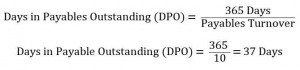STEP 4: CALCULATE THE CASH CONVERSION CYCLE ( CCC)

The final step is to sum up the resulting inventory, receivables, and payables conversion periods, calculated above: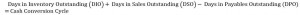Alternatively, we can express the above relationship using the turnover ratios we determined: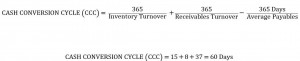It takes on average approximately 60 days for the company to inventory purchase, pay its suppliers, collect its receivables, and receive the cash

INTERPRETATION AND ANALYSIS

A low CCC is conducive to healthy working capital levels, profitability, liquidity, cash flows, and stable operating cycles.

From the example above, it takes approximately 60 days to convert inventory purchases into actual cash receipts. Needless to say, a short cash conversion cycle is desirable, and generally promotes healthy working capital levels and liquidity, cash flows, profitability, and stable operating cycles.

Understanding a company’s cash conversion cycle requires an examination of its 3 working capital accounts: accounts receivable, inventory, and accounts payable.  Managing the CCC involves managing the receivables, inventory, and payables functions.

Accounts Receivable

While an increase in receivables generates an increase in sales, holding receivables for a long time also ties up cash (since cash is received only when receivables are actually paid. Therefore, companies have an incentive to reduce the length of time that their receivables are outstanding. We can see this from the example of the equations above. Lower (or decreasing) outstanding receivables increase the receivables turnover ratio, which translates in a faster receivables conversion period (or a lower DSO). This in turn translates into a lower CCC.

Inventory

The same relationship applies to inventory. Decreasing the amount of time that inventories are held increases the inventory turnover ratio, which in turn decreases the inventory conversion period (or DIO). The effect is a lowering of the cash conversion cycle, an advantageous outcome.

Accounts Payable

The above causal relationships are reversed when dealing with payables. Companies have an incentive to lengthen the amount of time it takes to pay down payables, since that frees up and provides cash now.  Paying down payables requires the usage of cash, while an increase in payables from one period to the next increases cash.  From above, we see that higher average payables would lower the payables turnover ratio, and increases the payables conversion period (or DPO). Since the DPO is a negative component in the CCC equation, a higher DPO translates into a lower CCC – which is good.

CONCLUSION

Measuring the cash conversion cycle is important to liquidity, working capital, and the operating cycle of a company. Good management of the CCC can also enhance a company’s cash flows, allowing it to effectively make sound investing and financing decision. Managing the CCC entails efficient inventory, receivables, and payables functions, and should be part of a company’s overall operational strategy.

Keywords: Inventory Management, Fundamental Analysis, Accounts Payable, Accounts Recievable